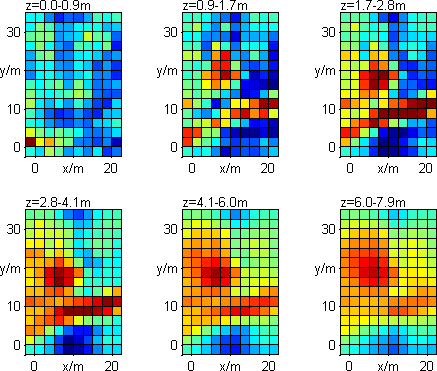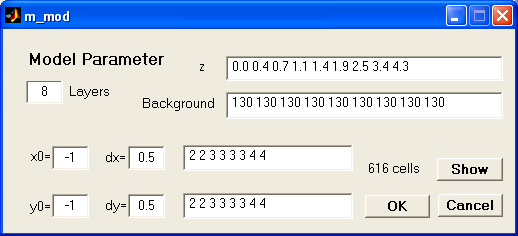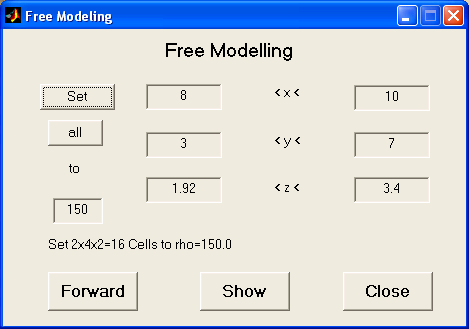Start Page Menu Items Interactive Features Hotkeys Tutorial

### Model parameter

This dialog is used to control the model parameterizations. There are two different parameter strategiesGRID MODEL: The model cells are orthogonal hexaedra bounded by lines of x, y and z nodes. In this dialog all cell boundaries can be defined seperately. By changing dx or dy a new (equidistant) vector is constructed. By changing the number of layers and pressing button 1dsens the layer boundaries are determined using 1d-sensitivity studies of the whole data set. By editing background resistivities for each layer a general layered model can be set. Note that the length of background and z vector are equal since the last layer is going to infinity. Those background values affect the fd forward result of the model.
 PARA MODEL: In this model type, the length and width can differ for different layers corresponding to different resolution properties. In the dialog the number of layers, the layer boundaries and its resistivities can be changed the same way like for grid model. The lower left boundary of the model is depicted by x0 and y0. By defining dx and dy the finest grid size in x and y direction is given, defaulting to one half of electrode spacing. The values nx and ny denote for each layer, how big the cells are, in multiples of dx and dy, respectively.### Free model edit

For setting up whole volumes to distinct resistivities the following dialog is useful.By typing boundaries for x, y and z the region can be limited and set to the desired resistivity value. The forward modelling can be started to compare data and model response.

### ASCII output of model

To save the model it can be exported into an column-oriented textfile, named like the datafile with extension *.mod, in the following format, one row for each model cell.

```x_1 x_2 y_1 y_2 z_1 z_2 resistivity
... ```

### ASCII input of model

The exported model (e.g. a synthetic model) can be loaded from file into workspace.

### Export vtk file

The model can be exported to a vtk (Visual Toolkit) file, which can be viewed with the free and powerful 3d viewer Paraview